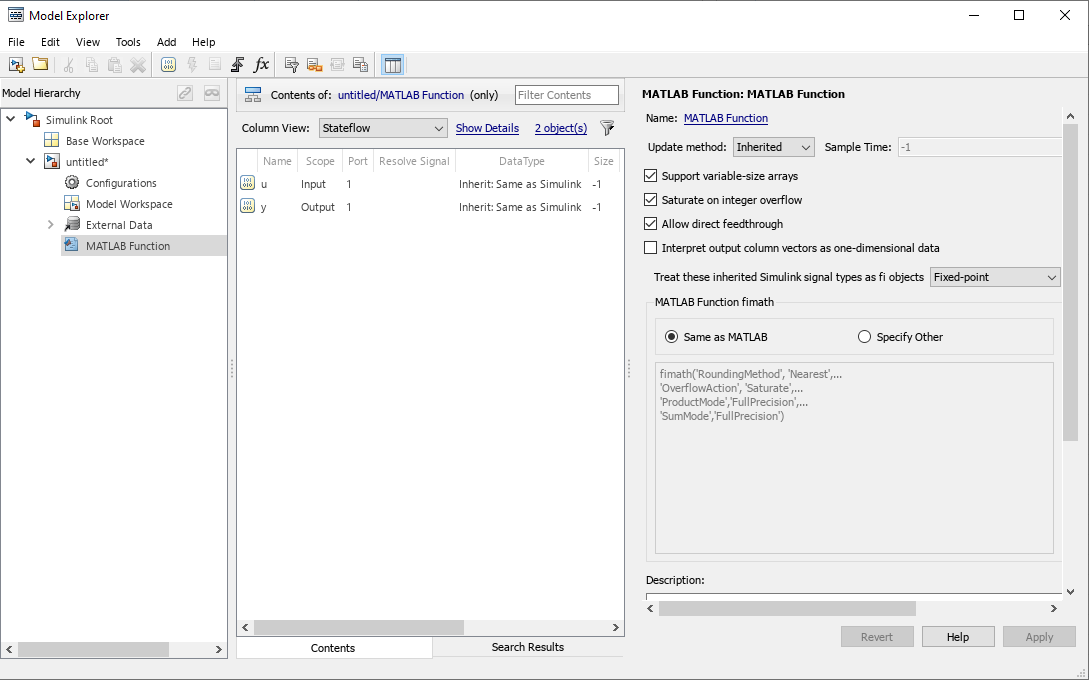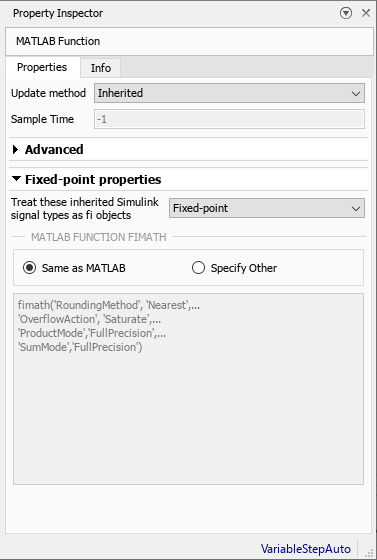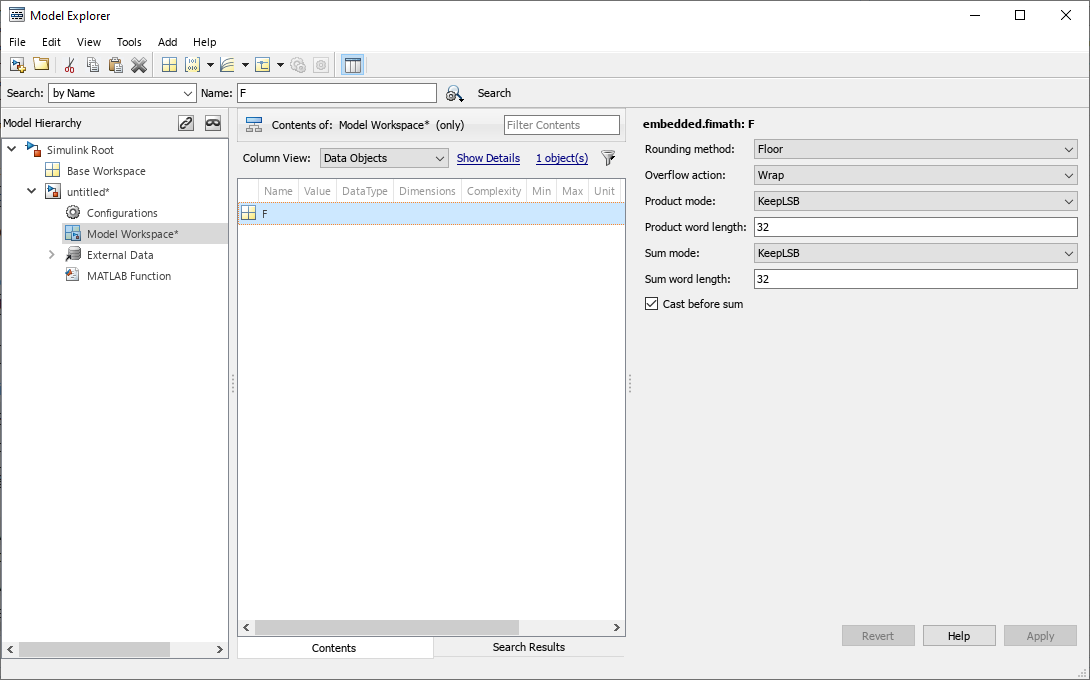## Code Generation with MATLAB Function Block

### Composing a MATLAB Language Function in a Simulink Model

The MATLAB Function block lets you compose a MATLAB® language function in a Simulink® model that generates embeddable code. When you simulate the model or generate code for a target environment, a function in a MATLAB Function block generates efficient C/C++ code. This code meets the strict memory and data type requirements of embedded target environments. In this way, the MATLAB Function blocks bring the power of MATLAB for the embedded environment into Simulink.

### MATLAB Function Block with Data Type Override

When you use the MATLAB Function block in a Simulink model that specifies data type override, the block determines the data type override equivalents of the input signal and parameter types. The block then uses these equivalent values to run the simulation. The following table shows how the MATLAB Function block determines the data type override equivalent using

• The data type of the input signal or parameter

• The data type override settings in the Simulink model

For more information about data type override, see `fxptdlg`.

Input Signal or Parameter TypeData Type Override SettingData Type Override Applies To SettingOverride Data Type
Inherited `single``Double``All numeric types` or `Floating-point`Built-in `double`
`Single``All numeric types` or `Floating-point`Built-in `single`
`Scaled double``All numeric types` or `Floating-point``fi scaled double`
Specified `single``Double``All numeric types` or `Floating-point`Built-in `double`
`Single``All numeric types` or `Floating-point`Built-in `single`
`Scaled double``All numeric types` or `Floating-point``fi scaled double`
Inherited `double``Double``All numeric types` or `Floating-point`Built-in `double`
`Single``All numeric types` or `Floating-point`Built-in `single`
`Scaled double``All numeric types` or `Floating-point``fi scaled double`
Specified `double``Double``All numeric types` or `Floating-point`Built-in `double`
`Single``All numeric types` or `Floating-point`Built-in `single`
`Scaled double``All numeric types` or `Floating-point``fi scaled double`
Inherited `Fixed``Double``All numeric types` or `Fixed-point``fi double`
`Single``All numeric types` or `Fixed-point``fi single`
`Scaled double``All numeric types` or `Fixed-point``fi scaled double`
Specified `Fixed``Double``All numeric types` or `Fixed-point``fi double`
`Single``All numeric types` or `Fixed-point``fi single`
`Scaled double``All numeric types` or `Fixed-point``fi scaled double`

For more information about using the MATLAB Function block with data type override, see Using Data Type Override with the MATLAB Function Block.

### Fixed-Point Data Types with MATLAB Function Block

Code generation from MATLAB supports a significant number of Fixed-Point Designer™ functions. Refer to Functions Supported for Code Acceleration or C Code Generation for information about which Fixed-Point Designer functions are supported.

Note

To simulate models using fixed-point data types in Simulink, you must have a Fixed-Point Designer license.

#### Specifying Fixed-Point Parameters in the Model Explorer

You can specify parameters for a MATLAB Function block in a fixed-point model using the Model Explorer. Try the following exercise:

1. Place a MATLAB Function block in a new model. You can find the block in the Simulink User-Defined Functions library.

2. Open the Model Explorer. On the Modeling tab, click Model Explorer.

3. Expand the untitled* node in the Model Hierarchy pane of the Model Explorer. Then, select the MATLAB Function node. The Model Explorer now appears as shown in the following figure.The following parameters in the Dialog pane apply to MATLAB Function blocks in models that use fixed-point and integer data types:

Treat these inherited Simulink signal types as fi objects

Choose whether to treat inherited fixed-point and integer signals as `fi` objects.

• When you select `Fixed-point`, the MATLAB Function block treats all fixed-point inputs as Fixed-Point Designer `fi` objects.

• When you select `Fixed-point & Integer`, the MATLAB Function block treats all fixed-point and integer inputs as Fixed-Point Designer `fi` objects.

MATLAB Function block fimath

Specify the `fimath` properties for the block to associate with the following objects:

• All fixed-point and integer input signals to the MATLAB Function block that you choose to treat as `fi` objects.

• All `fi` and `fimath` objects constructed in the MATLAB Function block.

You can select one of the following options for the MATLAB Function block fimath:

• Same as MATLAB — When you select this option, the block uses the same `fimath` properties as the current default fimath. The edit box appears dimmed and displays the current default fimath in read-only form.

• Specify Other — When you select this option, you can specify your own `fimath` object in the edit box.

For more information on these parameters, see Using fimath Objects in MATLAB Function Blocks.

#### Using fimath Objects in MATLAB Function Blocks

The MATLAB Function block fimath parameter enables you to specify one set of `fimath` object properties for the MATLAB Function block. The block associates the `fimath` properties you specify with the following objects:

• All fixed-point and integer input signals to the MATLAB Function block that you choose to treat as `fi` objects.

• All `fi` and `fimath` objects constructed in the MATLAB Function block.

You can set these parameters on the following dialog box, which you can access through the Ports and Data Manager.• To access this pane through the Model Explorer:

• On the Modeling tab, click Model Explorer.

• Then, select the MATLAB Function block from the Model Hierarchy pane on the left side of the Model Explorer.

• To access this pane through the Ports and Data Manager, on the MATLAB Editor tab, clickEdit Data.

When you select Same as MATLAB for the MATLAB Function block fimath, the MATLAB Function block uses the current default fimath. The current default fimath appears dimmed and in read-only form in the edit box.

When you select Specify other the block allows you to specify your own `fimath` object in the edit box. You can do so in one of two ways:

• Constructing the `fimath` object inside the edit box.

• Constructing the `fimath` object in the MATLAB or model workspace and then entering its variable name in the edit box.

Note

If you use this option and plan to share your model with others, make sure you define the variable in the model workspace. See Sharing Models with Fixed-Point MATLAB Function Blocks for more information on sharing models.

The Fixed-Point Designer `isfimathlocal` function supports code generation for MATLAB.

#### Sharing Models with Fixed-Point MATLAB Function Blocks

When you collaborate with a coworker, you can share a fixed-point model using the MATLAB Function block. To share a model, make sure that you move any variables you define in the MATLAB workspace, including `fimath` objects, to the model workspace. For example, try the following:

1. Place a MATLAB Function block in a new model. You can find the block in the Simulink User-Defined Functions library.

2. Define a `fimath` object in the MATLAB workspace that you want to use for any Simulink fixed-point signal entering the MATLAB Function block as an input:

```F = fimath('RoundingMethod','Floor','OverflowAction','Wrap',... 'ProductMode','KeepLSB','ProductWordLength',32,... 'SumMode','KeepLSB','SumWordLength',32) F = RoundingMethod: Floor OverflowAction: Wrap ProductMode: KeepLSB ProductWordLength: 32 SumMode: KeepLSB SumWordLength: 32 CastBeforeSum: true```

3. Open the Model Explorer. On the Modeling tab, click Model Explorer.

4. Expand the untitled* node in the Model Hierarchy pane of the Model Explorer, and select the MATLAB Function node.

5. Select Specify other for the MATLAB Function block fimath parameter and enter the variable `F` into the edit box on the Dialog pane. Click to save your changes.

You have now defined the `fimath` properties to be associated with all Simulink fixed-point input signals and all `fi` and `fimath` objects constructed within the block.

6. Select the Base Workspace node in the Model Hierarchy pane. You can see the variable `F` that you have defined in the MATLAB workspace listed in the Contents pane. If you send this model to a coworker, that coworker must first define that same variable in the MATLAB workspace to get the same results.

7. Cut the variable `F` from the base workspace, and paste it into the model workspace listed under the node for your model, in this case, untitled*. The Model Explorer now appears as shown in the following figure.You can now email your model to a coworker. Because you included the required variables in the workspace of the model itself, your coworker can simply run the model and get the correct results. Receiving and running the model does not require any extra steps.

## Support